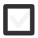# Calculate the mean, e.g. of numbers 17, 11, 8. Can estimate length in everyday situations.+1 vote
163 views

## 1 lesson

by Guru (5.9k)
edited
Through out the year you might get different grades in school. Some grades could be excellent some not so good. At the end of the semester you might want to calculate your average grade. How can you do this?

If we would like to find a middle between two number values le'ts say 5 and 3 all we need to do is to find mean or average.

In order to do that we should add up the numbers and devide the sum with 2.

(5 + 3) ÷ 2 = 8 ÷ 2 = 4

Now 4 is exactly between 5 and 3 and it is the average value, of the two numbers, equally distant from both. Finding an average of 5 and 3 was easy, you could do it even without calculation, but what if you want to calculate of average of more than two numbers?

Well in the first example we added up the numbers and divied the sum with two and two is the amount of number values we added. This means if we would like to find an average for more than two numbers we would also have to add them up and devide the sum but with the value equal to the how many numbers we added.

As an example we can find the average of 17,11,8.

(17 + 11 + 8) ÷ 3 = 36 ÷ 3 = 12

So we first added up the numbers and because we added three numbers, we devided the sum with three and we got an average or a mean of twelve.

Twelve is not equally distant from all three numbers, but if we would share the sum of all numbers equally this is the number we get which makes it kind of the "central" value for these numbers.

No matter how many values we have if we want to find the mean value we only need to add them all and devide the summ with a number equal to how many values we added.

To find out more you can check out these interesting videos that do more examples on average values.

This interestig video shows the practicall application of calculating and understanding average:

Not all average values are integer numbers check out this video for a none integer average.